# Area of a Trapezium

In this worksheet students will learn the formula for finding the area of a trapezium and use it to calculate areas.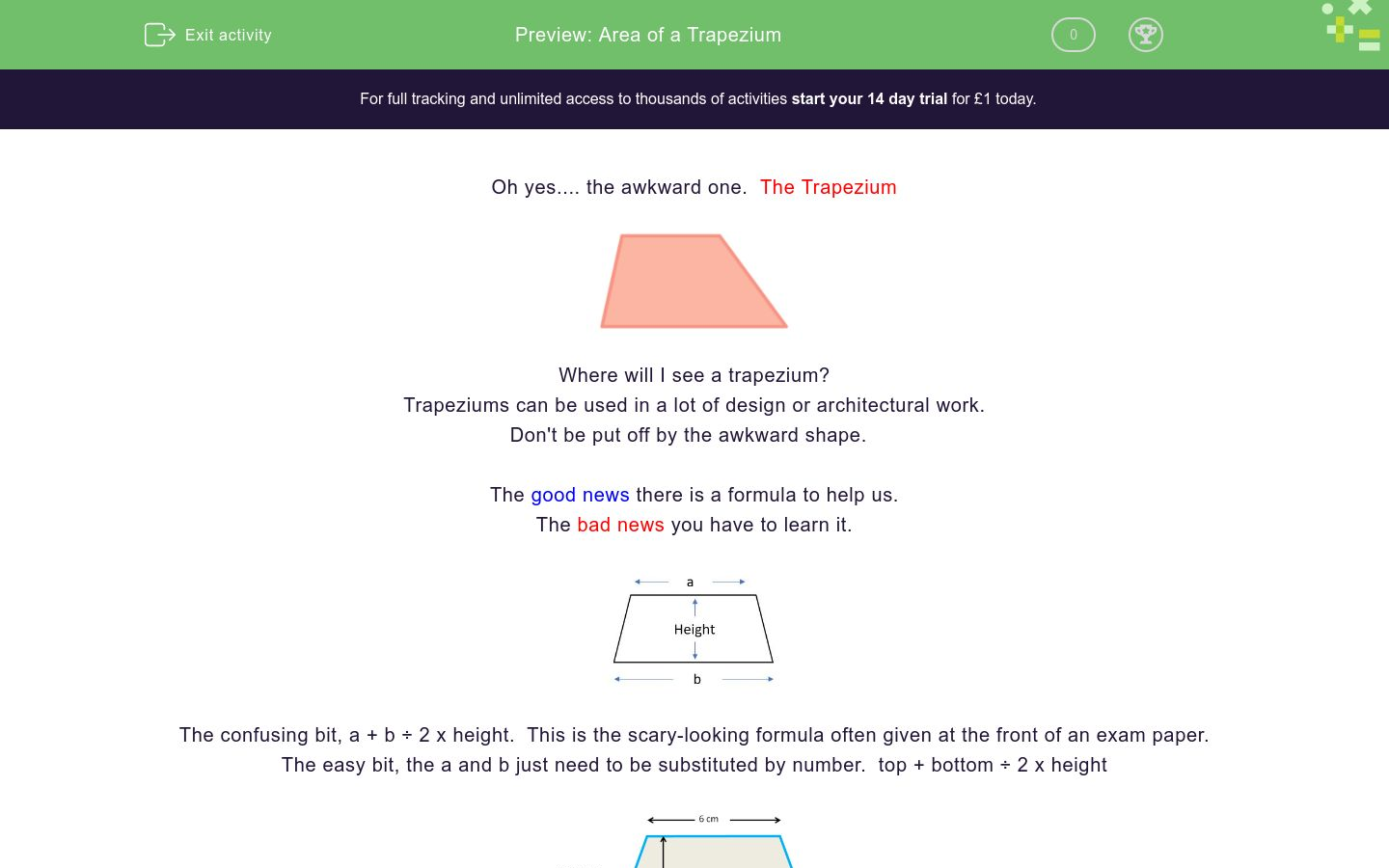Key stage:  KS 4

GCSE Subjects:   Maths

GCSE Boards:   AQA, Eduqas, Pearson Edexcel, OCR

Curriculum topic:   Geometry and Measures, Mensuration

Curriculum subtopic:   Mensuration and Calculation, Area Calculations

Difficulty level:### QUESTION 1 of 10

Oh yes.... the awkward one.  The TrapeziumWhere will I see a trapezium?

Trapeziums can be used in a lot of design or architectural work.

Don't be put off by the awkward shape.

The good news there is a formula to help us.

The bad news you have to learn it.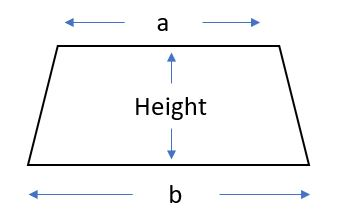The confusing bit  a + b ÷ 2 x height.  This is the scary looking formula often given at the front of an exam paper.

The easy bit  the a and b just need to be substituted by number.  top + bottom ÷ 2 x height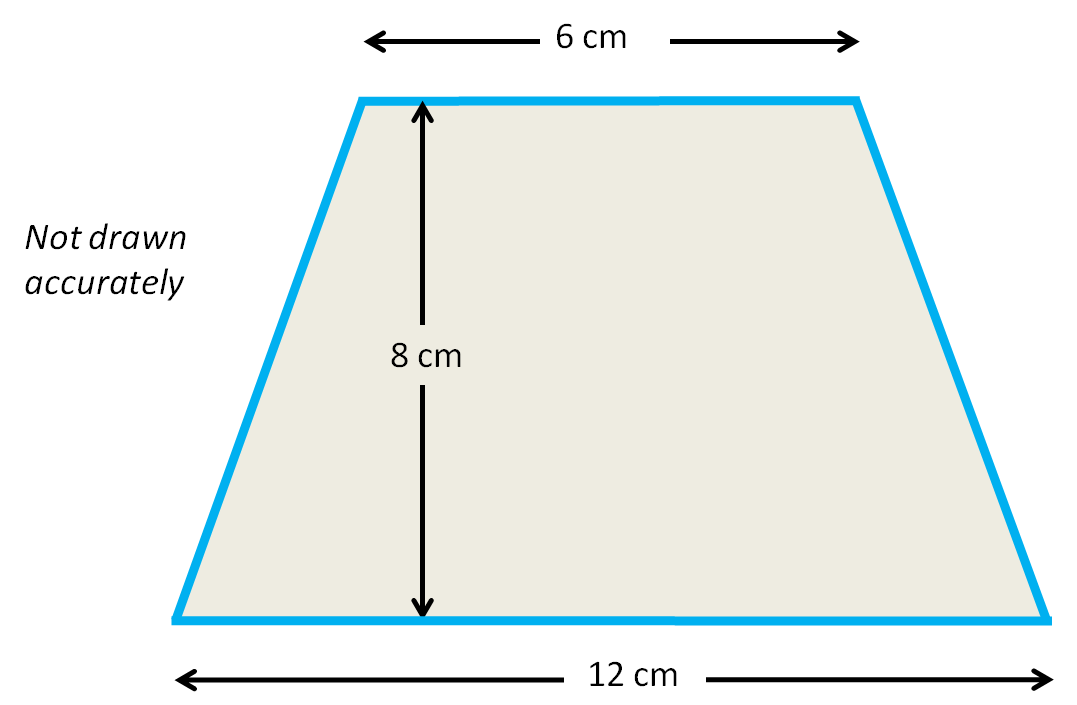Area of this trapezium is 6 + 12 = 18  18 ÷ 2 = 9    9 x 8 = 72 cm²

This will soon become second nature.

Remember when working with area we always answer in units squared.Now you could go barking up the wrong tree so be careful.

When you are given measurements for a trapezium you are sometimes given the top, bottom, vertical height and a slanted height.

You always want the vertical height as shown in the diagram above.

Once you have learned the formula you are flying.Find the area of this trapezium.

320 m²

240 m²

360 m²

160 m²

Match these areas

## Column B

Trapezium top 4 cm bottom 3cm , height 5 cm
20 cm²
Trapezium top 6 cm bottom 8 cm , height 4 cm
28 cm²
Trapezium top 9 cm bottom 3cm , height 4 cm
24 cm²
Trapezium top 5 cm, bottom 3 cm , height 5 cm
0.2 cm²
Trapezium top 0.4 cm bottom 0. 8 cm , height 0.25 ...
0.15 cm²
Trapezium top 0.3 cm bottom 0.7cm , height 0.4 cm
17.5 cm²Find the area of this trapezium.

Round to 1 decimal place (1 d.p)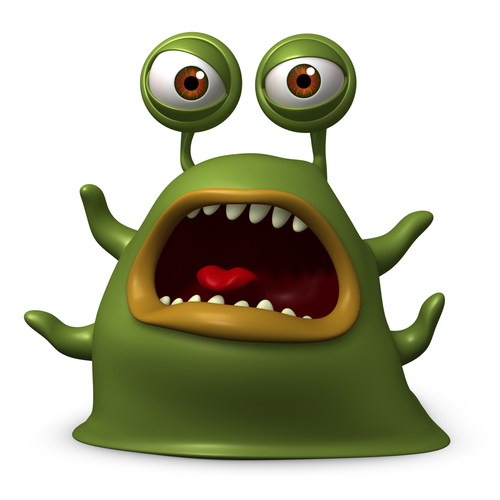This aliens mouth is trapezium shaped.

What is the area of one triangle of his mouth if the top lip measures 10 cm and the bottom lip is 12 cm?

When his mouth is open, the distance between the top lip and bottom lip is 6 cm.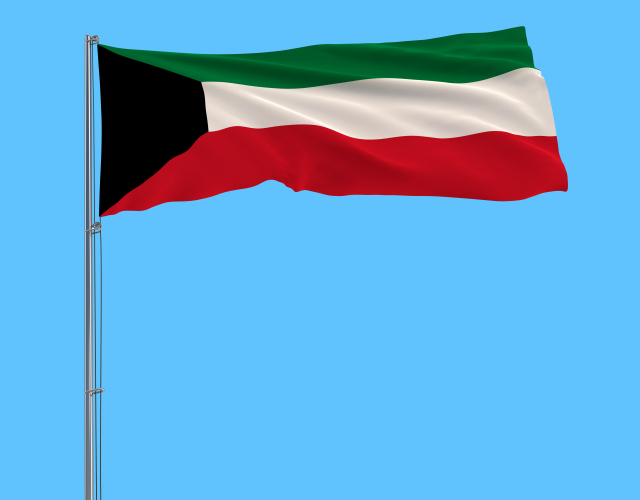This trapezium in the flag of Kuwait has the following measurements.

Bottom 0.6 m, top 0.3 m  height of 40 cm.  Calculate its area.

0.18 m²

1.8 m²

1800 cm²

180 cm²This face of this trapezium block has the following measurements.  Top 4 cm, bottom 6 cm and height 9 cm

A second blocks measurements are double.

What is the difference in area between the faces of the two trapeziums?

125 cm²

135 cm²

200 cm²

50 cm²A trophy base has as base of  30 cm, the top measures  25 cm  and a height of 15 cm.

What is the area of the face of the trophy base?

15 of these bases are needed for a a presentation.  What is the total area of metal used in making 15 of these faces?The base of this trapezium measures x and top measures the height is x + 4.  The height is 5 cm. What is its area?

2x + 4; ÷ 2 x 5 cm²

x² + 4; ÷ 2 x 5 cm²

2x + 10; ÷ 2 x 5 cm²

x² + 4; ÷ 2 x 5 cm²The base of this trapezium measures 2x  + 3, the top measures 3x - 1  and the height is x + 2.

What are the two ways you can write its area?

2x + 3 + 3x - 1 ÷ 2 multiplied by x + 2

5x + 4 ÷ 2 multiplied by x + 2

2x + 4+ 3x - 1 ÷ 2 multiplied by x + 2

5x + 2 ÷ 2 multiplied by x + 2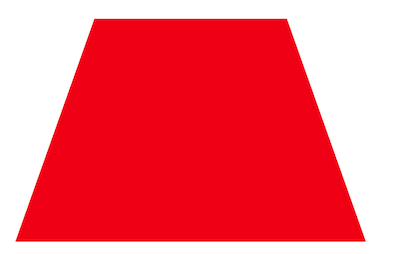The area of this trapezium is 42 cm²  If the top is 6 cm and the bottom is 8 cm, calculate the height.

• Question 1Find the area of this trapezium.

160 m²
EDDIE SAYS
Did you apply the formula? top + bottom ÷ 2 then x by height 12 x 20 = 32 m² 32m² ÷ 2 = 16 m² 16 x 10 = 160 m² It is easy to forget the steps so keep practising
• Question 2

Match these areas

## Column B

Trapezium top 4 cm bottom 3cm , h...
17.5 cm²
Trapezium top 6 cm bottom 8 cm , ...
28 cm²
Trapezium top 9 cm bottom 3cm , h...
24 cm²
Trapezium top 5 cm, bottom 3 cm ,...
20 cm²
Trapezium top 0.4 cm bottom 0. 8 ...
0.15 cm²
Trapezium top 0.3 cm bottom 0.7cm...
0.2 cm²
EDDIE SAYS
Hopefully after this activity applying the formula is now getting easy. Keep remembering the divide by 2. If you forget throughout the activity make sure you do it at the end. Top + bottom, divide by two x height you will be saying it in your sleep. 4 + 3 ÷ 2 x 5 = 17.5 cm² 6 + 8 ÷ 2 x 4 = 28 cm² 9 + 3 ÷ 2 x 4 = 24 cm² 5+ 3 ÷ 2 x 5 = 20 cm² 0.4 + 0.8 ÷ 2 x 0.25= 0.15 cm² 0.3 + 0.7 ÷ 2 x 0.4 = 0.2 cm²
• Question 3Find the area of this trapezium.

Round to 1 decimal place (1 d.p)

92.4
EDDIE SAYS
Don't be frightened of decimals. Just make sure you round to the correct number of decimal places. 6.5 +15.5 ÷ 2 = 11 cm² x 8.4 cm = 92.4 cm²
• Question 4This aliens mouth is trapezium shaped.

What is the area of one triangle of his mouth if the top lip measures 10 cm and the bottom lip is 12 cm?

When his mouth is open, the distance between the top lip and bottom lip is 6 cm.

66 cm²
EDDIE SAYS
Okay, so a slightly different way of interpreting a trapezium. Just to see you haven't fallen asleep looking at basic trapeziums. 10 + 12 = 22 ÷ 2 = 11 cm x 6 = 66 cm² I think this alien looks more shocked than scary.
• Question 5This trapezium in the flag of Kuwait has the following measurements.

Bottom 0.6 m, top 0.3 m  height of 40 cm.  Calculate its area.

0.18 m²
1800 cm²
EDDIE SAYS
Did you spot the two different units? Examiners like to sneak these in. You can convert them either way 0.6 + 0.3 = 0.9 m ÷2 = 0.45 x 0.4 = 0.18 cm² 60 + 30 = 90 cm ÷2 = 45 cm x 40 = 1800 cm²
• Question 6This face of this trapezium block has the following measurements.  Top 4 cm, bottom 6 cm and height 9 cm

A second blocks measurements are double.

What is the difference in area between the faces of the two trapeziums?

135 cm²
EDDIE SAYS
This is just a 3 step question. You have all the knowledge that you need. Area of original trapezium = 4 + 6 ÷ 2 x 5 = 45 cm² Area of second trapezium = 8 + 12 ÷ 2 x 10 = 180 cm² 180 - 45 = 135 cm²
• Question 7A trophy base has as base of  30 cm, the top measures  25 cm  and a height of 15 cm.

What is the area of the face of the trophy base?

15 of these bases are needed for a a presentation.  What is the total area of metal used in making 15 of these faces?

412.5
EDDIE SAYS
You have to be careful to ready the question carefully. You are only asked for the face of the trophy base. So look at that part only. 30 + 25 ÷2; 27.5 x 15 = 412.5 cm² 1200 ÷ 2 = 600 600 x 10 = 6000 cm²
• Question 8The base of this trapezium measures x and top measures the height is x + 4.  The height is 5 cm. What is its area?

2x + 4; ÷ 2 x 5 cm²
EDDIE SAYS
A curved ball, don't you just love them In some ways these are easier because you don't know the value of x. All you can do is write what you know X + 4 + x = 2x + 4 2x + 4 ÷ 2 x 5 cm²
• Question 9The base of this trapezium measures 2x  + 3, the top measures 3x - 1  and the height is x + 2.

What are the two ways you can write its area?

2x + 3 + 3x - 1 ÷ 2 multiplied by x + 2
5x + 2 ÷ 2 multiplied by x + 2
EDDIE SAYS
And another just for fun. In some ways these are easier because you don't know the value of x. All you can do is write what you know so (2x + 3) + (3x - 1) ÷ 2 x (x + 2) or you may simplify it to 5x + 2 ÷ 2 x (x + 2) Brackets just help to separate things as using x and the multiplication sign can get confusing.
• Question 10The area of this trapezium is 42 cm²  If the top is 6 cm and the bottom is 8 cm, calculate the height.

6 cm
EDDIE SAYS
Okay just when you thought you had everything under control. You have all the information you need. Area = top + bottom ÷2 x height Substitute what you know into the formula 42 cm² = 6 + 8 ÷2 = 7 Work backwards 42 ÷ 7 = 6 Test it out! 6 + 8 ÷ 2 x 6 = 42 cm²
---- OR ----

Sign up for a £1 trial so you can track and measure your child's progress on this activity.

### What is EdPlace?

We're your National Curriculum aligned online education content provider helping each child succeed in English, maths and science from year 1 to GCSE. With an EdPlace account you’ll be able to track and measure progress, helping each child achieve their best. We build confidence and attainment by personalising each child’s learning at a level that suits them.

Get started